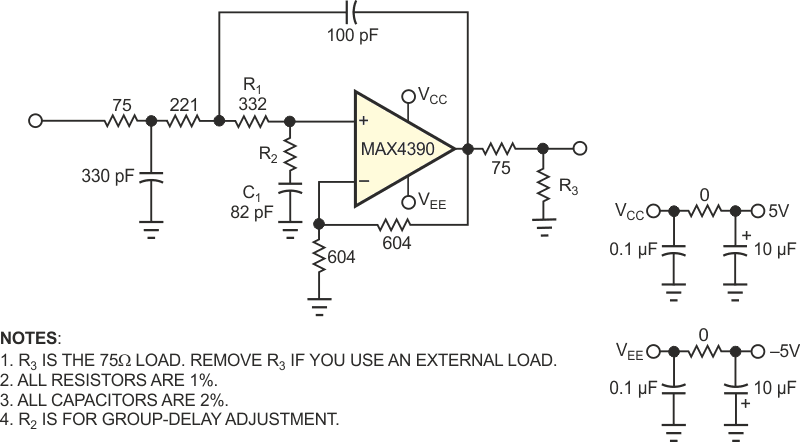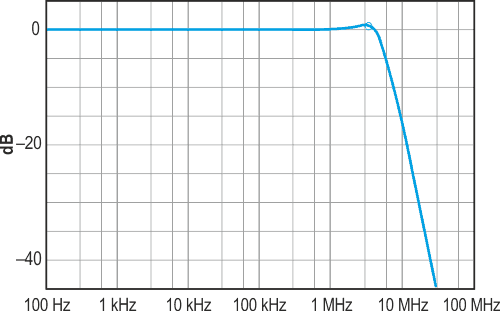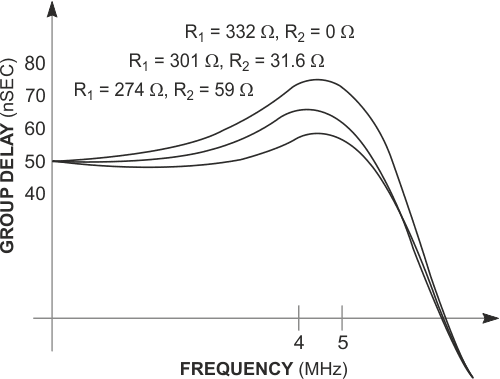# Butterworth filter has adjustable group delay

## Maxim MAX4392

The Sallen-Key realization of a 5.25-MHz, three-pole Butterworth filter has a gain of 2 V/V and can drive 75 Ω back-terminated coax with an overall gain of 1 (Figure 1). Used to reconstruct component-video (Y, Pb, Pr) and RGB signals, this filter has an insertion loss greater than 20 dB at 13.5 MHz and greater than 40 dB at 27 MHz (Figure 2). Like the antialiasing filter before an ADC, this filter removes the higher frequency replicas of a signal following a DAC. To preserve quality in the video waveform, you should minimize group-delay variations in the filter and any group-delay differential between filters. That requirement mandates a means for adjusting the filter's group delay without affecting its bandwidth. In Figure 1, the addition of R2 in series with C1 and R1 creates a lag-lead network.Figure 1. This three-pole Butterworth video-reconstruction filter has adjustable group delay.Figure 2. The typical filter response for the circuit of Figure 1 is R1 + R2 = 332 Ω.

Keeping the sum of R1 and R2 constant and equal to the original R1 value preserves bandwidth by preserving the dominant-pole frequency. Increasing the R1 value, on the other hand, introduces a “lead” term that lowers group delay by reducing the rate of change in phase. For R2 = 0 Ω and R1 = 332 Ω in the circuit shown, the average group-delay variation over the filter bandwidth is about 25 nsec. Raising R2 to 31.6 Ω and lowering R1 to 301 Ω decreases the variation to approximately 15 nsec, and setting R2 =59 Ω with R1 =274 Ω decreases it to approximately 7 nsec. The last case has a less-than 0.5-dB effect on band-edge selectivity but does not change the filter's 3-dB bandwidth (Figure 3).Figure 3. Selected values of R1 and R2 allow control of group-delay variation over the filter’s passband.

EDN